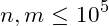# CF1009G Allowed Letters 题解,G中有一组无公共点的边，一端恰好为组成X的点的充分必要条件是：X中的任意k个点至少与Y中的k个点相邻。(1≤k≤m)。

// Author : WangZhikun
// Date   : 2018.07.27

#include <cstdio>
#include <vector>
#include <cstring>
#include <iostream>
#include <algorithm>

using namespace std;

int n,m,msk,p,fn = {0},gn = {0},ccnt = {0};
char s,cm,ans;

int main() {
ios::sync_with_stdio(false);
cin.tie(0);
for(int i=0;i<100010;i++)msk[i] = 63;
cin>>s;
n = (int)strlen(s);
cin>>m;

for(int i=0;i<m;i++){
cin>>p>>cm;
p-=1;
msk[p] = 0;
for(int i=0;i<strlen(cm);i++)
if(cm[i]>='a' && cm[i]<='f')
msk[p]+=1<<(cm[i]-'a');
}
for(int i=0;i<n;i++){
fn[msk[i]]++;
ccnt[s[i]-'a']+=1;
}
for(int i=0;i<64;i++){
for(int j=0;j<64;j++)if((i&j) == j)gn[i]+=fn[j];
}
for(int po=0;po<n;po++){
ans[po] = 'u';
for(int i=0;i<64;i++)if((i&msk[po]) == msk[po])gn[i]-=1;
for(int c = 0;c<6;c++){
if((msk[po] & (1<<c)) == 0)continue;
ccnt[c]-=1;
int co = 1,ccn = 0;
for(int i=0;i<64;i++){
ccn = 0;
for(int j=0;j<6;j++)if(i&(1<<j))ccn+=ccnt[j];
if(ccn<gn[i]){
co = 0;
break;
}
}
if(co){
ans[po] = 'a'+c;
break;
}
else{
ccnt[c]+=1;
}
}
if(ans[po] == 'u'){
cout<<"Impossible"<<endl;
return 0;
}
}
cout<<ans<<endl;
return 0;
}

[latex]